# Anisotropy analysis by IMT – 3D

In three dimensions, irreducible Minkowski tensors (IMT’s) are defined by an expansion of the density of surface normal directions, in complete analogy to the two-dimensional case. The distribution of surface normals uniquely determines a convex object. (This statement is known as Minkowski’s problem. In this context, the surface normal density is sometimes called the extended Gaussian image. )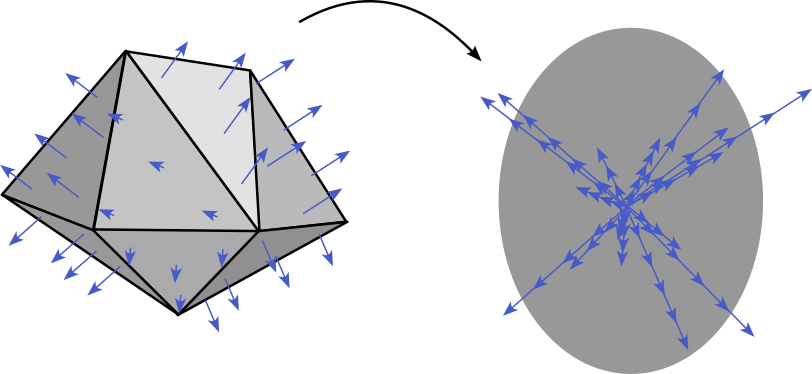It is useful to select the complete orthogonal set of Spherical harmonics as basis functions. For a polyhedron with face areas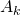and normals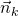, the IMT’s are given by.

Here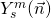is the obvious notation for the spherical harmonic evaluated with the direction corresponding to the normal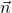. The index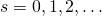labels increasing tensor ranks, and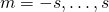is the “magnetic quantum number”. The complex coefficients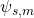have the usual transformation properties of spherical harmonics under rotations.

Often, it is useful to define rotational invariants which can serve as fingerprints of a body irrespective of its orientation in space.  The quadratic invariants in particular are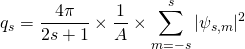.

For convenience, we have also divided by the total surface area, such that the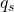are invariant under translation, rotation, and scaling.

Three-dimensional irreducible Minkowski Tensors are discussed in greater detail in Ref. . When computed for Voronoi cells, they generalize Paul Steinhardt’s bond-orientational order parameters .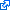B. K. Horn, “Extended Gaussian images,” Proceedings of the IEEE, vol. 72, iss. 12, p. 1671–1686, 1984.W. Mickel, S. C. Kapfer, G. E. Schröder-Turk, and K. Mecke, “Shortcomings of the bond orientational order parameters for the analysis of disordered particulate matter,” Journal of Chemical Physics, vol. 138, iss. 4, p. 44501, 2013.P. J. Steinhardt, D. R. Nelson, and M. Ronchetti, “Bond-orientational order in liquids and glasses,” Phys. Rev. B, vol. 28, p. 784–805, 1983.